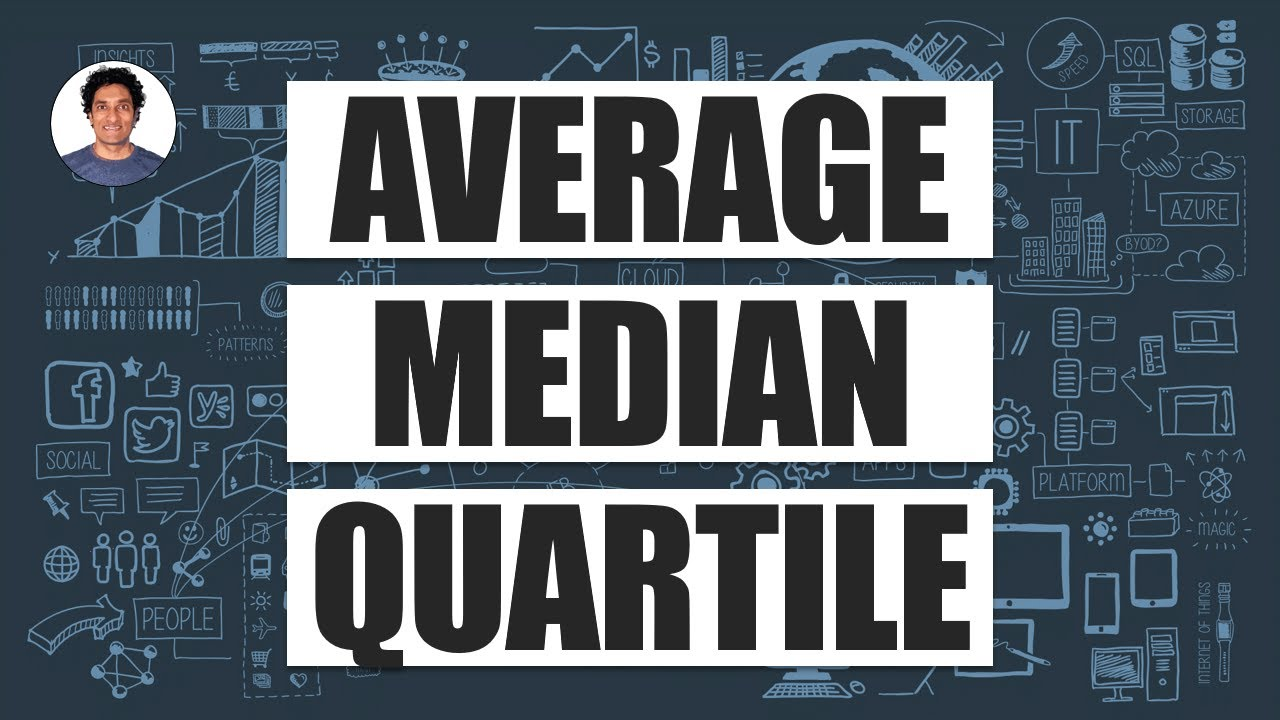en | deNov 9, 2022 12:00 AM

# AVERAGE vs. MEDIAN - Which is better?

## AVERAGE, MEDIAN, QUARTILE - do you know the difference?In this simple but effective explanation understand what these are and how & when to use them with you...

AVERAGE, MEDIAN, QUARTILE - do you know the difference?

In this simple but effective explanation understand what these are and how & when to use them with your data. And yeah, Bill Gates walks into a bar...

## In this Video

0:00 - Bill Gates walks into a bar (the Average problem)

0:59 - AVERAGE Explained

2:12 - Calculating AVERAGE in Excel

2:40 - MEDIAN Explained (AVERAGE vs. MEDIAN)

4:27 - QUARTILES Explained

5:53 - Important Rule when working with data (always see the data)

7:30 - How to apply these ideas in work

• Mean is calculated by dividing the sum of the data values by the number of data values.
• Median is the middle data value, at 50% of the data, where there is an equal probability of data values falling below or above it.
• The lower/first quartile is at 25% of the data, or the middle between of the lower half of data.
• The upper/third quartile is at 75% of the data, or the middle between the upper half of data.

Median, Quartiles And Percentiles (Ungrouped Data)

In these lessons, we will learn how to find the median, quartiles and percentiles of ungrouped data (discrete data).

https://www.onlinemathlearning.com/quartile.html

HubSite 365 Apps